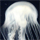## Send to a Friend#### What is the name of the theorem that proves this equation?

The equation is as follows:

a^3—b^3 = 3(b^2) + 3b + 1
ex) 10^3—9^3 = 3(9^2) + 3(9) + 1 = 271

I saw it used on a math video. I am interested in how it is proved.Topics: ,

or

#### Using Email

Separate multiple emails with commas.
We’ll only use these emails for this message.

`